Disclaimer: This is an example of a student written essay.

Any opinions, findings, conclusions or recommendations expressed in this material are those of the authors and do not necessarily reflect the views of UKEssays.com.

# Curvelet-based Bayesian Estimator for Speckle Suppression

Info: 4482 words (18 pages) Essay
Published: 18th Aug 2017

Curvelet-based Bayesian Estimator for Speckle Suppression in Ultrasound Imaging

Abstract. Ultrasound images are inherently affected by speckle noise, and thus the reduction of this noise is a crucial pre-processing step for their successful interpretation. Bayesian estimation is a powerful signal estimation technique used for speckle noise removal in images. In the Bayesian-based despeckling schemes, the choice of suitable statistical models and the development of a shrinkage function for estimation of the noise-free signal are the major concerns. In this paper, a novel curvelet-based Bayesian estimation scheme for despeckling of ultrasound images is developed. The curvelet coefficients of the multiplicative degradation model of the noisy ultrasound image are additively decomposed into noise-free and signal-dependent noise components. The Cauchy and two-sided exponential distributions are assumed to be statistical models for the noise-free and signal-dependent noise components of the observed curvelet coefficients, respectively, and an efficient low-complexity realization of the Bayesian estimator is proposed. The experimental results demonstrate the validity of the proposed despeckling scheme in providing a significant suppression of the speckle noise and simultaneously preserving the image details.

Keywords:Ultrasound imaging, curvelet transform, speckle noise, Bayesian estimation, statistical modeling.

1. Introduction

Ultrasound imaging is important for medical diagnosis and has the advantages of cost effectiveness, port-ability, acceptability and safety . However, ultrasound images are of relatively poor quality due to its contamination by the speckle noise, which considerably degrades the image quality and leads to a negative impact on the diagnostic task. Thus, reducing speckle noise while preserving anatomic information is necessary to better delineate the regions of interest in the ultrasound images.

In the work of speckle suppression in ultrasound images, many spatial-based techniques that employ either single-scale or multi-scale filtering have been developed in the literature [2-4]. Earlydeveloped single-scale spatial filtering  are limited in their capability for significantly reducing the speckle noise. More promising spatial single-scale techniques such as those using bilateral filtering  and nonlocal filtering  have been recently proposed.This work was supported in part by the Natural Sciences and Engineering Research Council (NSERC) of Canada and in part by the Regroupement Strategique en Microelectronique du Quebec (ReSMiQ).

These techniques depend on the size of the filter window, and hence, for a satisfactory speckle suppression, they require large computational time. Alternatively, multi-scale spatial techniques , based on partial differential equations, have been investigated in the literature. These techniques are iterative and can produce smooth images with preserved edges. However, important structural details are unfortunately degraded during the iteration process. As an appropriate alternative to spatial-based speckle suppression in ultrasound images, many other despeckling techniques based on different transform domains, such as the ones of wavelet, contourlet, and curvelet, have been recently proposed in the literature [6-8]. Wavelet transform has a good reputation as a tool for noise reduction but has the drawback of poor directionality, which makes its usage limited in many applications. Using contourlet transform provides an improved noise reduction performance due to its property of fi‚exible directional decomposability. However, curvelet transform offers a higher directional sensitivity than that of contourlet transform and is more efficient in representing the curve-like details in images.

If you need assistance with writing your essay, our professional essay writing service is here to help!

For the development of despeckling techniques based on transform domains, thresholding  has been presented as a technique to build linear estimators of the noise-free signal coefficients. However, the main drawback of this thresholding technique is in the difficulty of determining a suitable threshold value. To circumvent this problem, non-linear estimators  have been statistically developed based on Bayesian estimation formalism. For the development of an efficient Bayesian-based despeckling scheme, the choice of a suitable probability distribution to model the transform domain coefficients is a major concern. Also, while investigating a suitable statistical model, the complexity of the Bayesian estimation process should be taken into consideration. Consequently, special attention should be paid to the realization complexity of the Bayesian estimator that results from employing the selected probabilistic model in one of the Bayesian frameworks.

In this paper, to achieve a satisfactory performance for despeckling of ultrasound images at a lower computational effort, a new curvelet-based Bayesian scheme is proposed. The multiplicative degradation model representing an observed ultrasound image is decomposed into an additive model consisting of noise-free and signal-dependent noise components. Two-sided exponential distribution is used as a prior statistical model for the curvelet coefficients of the signal-dependant noise. This model, along with the Cauchy distribution is used to develop a low-complexity Bayesian estimator. The performance of the proposed Bayesian despeckling scheme is evaluated on both syntheticallyspeckled and real ultrasound images, and the results are compared to that of some other existing despeckling schemes.

1. Modeling of Curvelet Coefficients

The multiplicative degradation model of a speckle-corrupted ultrasound image g(i,j) in the spatial domain is given by

g(i,j) = v(i,j)s(i,j)(1)

where v(i,j) and s(i,j) denote the noise-free image and the speckle noise, respectively. This model of the noisy observation of v(i,j) can be additively decomposed as a noise-free signal component and a signal-dependant noise:

g(i,j) = v(i,j) + (s(i,j) âˆ’1)v(i,j)

= v(i,j) + u(i,j)(2)

where (s(i,j) âˆ’1)v(i,j) represents the signal-dependant noise. Taking the curvelet transform of (2) at level l, we have

y[l,d](i,j) = x[l,d](i,j) + n[l,d](i,j)(3)

where y[l,d](i,j), x[l,d](i,j) and n[l,d](i,j) denote, respectively, the (i,j)th curvelet coefficient of the observed image, the corresponding noise free image and the corresponding additive signal-dependant noise at direction d= 1,2,3,Â·Â·Â·,D. In order to simplify the notation, we will henceforth drop both the superscripts land dand the index (i,j).

In this work, in order to reduce the noise inherited in ultrasound images, we propose exploiting the statistical characteristics of the curvelet coefficients in (3) to derive an efficient Bayesian estimator. Thus, one needs to provide a prior probabilistic model for the curvelet coefficients of xand

n. It has been shown that the distribution of the curvelet coefficients of noise-free images can be suitably modeled by the Cauchy distribution . The zero-mean Cauchy distribution is given by

px(x) = (Î³/Ï€)(x2 + Î³2)(4)

where Î³is the dispersion parameter. The noisy observation is used to estimate the Cauchy distribution parameter Î³by minimizing the function2

Â Ï†Ë†yyt

(t) âˆ’Ï†(t) eâˆ’

dt(5)

where Ï†Ë†y(t) is the empirical characteristic function corresponding to the curvelet coefficients yof

22the noisy observation, Ï†y(t) = Ï†x(t)Ï†E(t), Ï†x(t) = eâˆ’Î³|t|, and Ï†E(t) = eâˆ’(Ïƒï¿½/2)|t|

deviation ÏƒEobtained as

with the standard

ÏƒE=

0.6745

(6)

In (6), MAD denotes the median absolute deviation operation. Now, in order to formulate the Bayesian estimator, a prior statistical assumption for the curvelet coefficients of nof the signal dependant noise should also be assumed. From experimental observation, it is noticed that the tail part of the empirical distribution of ndecays at a low rate. Hence, in this paper, we propose to use a two-sided exponential (TSE) distribution given by

1

pn(n) =eâˆ’|n|/Î²

2Î²

(7)where Î²is a positive real constant referred to as the scale parameter. The method of log-cummulants (MoLC) is adopted to estimate the parameter Î², and thus the estimated Î²Ëœ is obtained by using the following expression:

Î²Ëœ = exp

1N1Â Â Â Â Â Â Â Â  N2

log(y(i,j))+ Î¾

(8)

N1N2

i=1j=1

where Î¾is the Euler-Mascheroni constant and N1 and N2 define the size N1 Ã-N2 of the curvelet subband considered.

1. Bayesian Estimator

Due to the fact that each of the Cauchy and TSE distributions has only one parameter, one could expect the process of Bayesian estimation to be of lower complexity. The values of the Bayes estimates xË† of the noise-free curvelet coefficients xof a subband under the quadratic loss function, which minimizes the mean square error (MSE), are given by the shrinkage function:

xË†(y) =px|y(x|y)xdx

P pn(yâˆ’x)px(x)xdx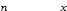=P p(yâˆ’x)p(x)

(9)

It is noted that a closed-form expression for

xË†(y) given by the above equation does not exist.

Thus, in order to obtain the Bayesian estimates for the noise-free curvelet coefficients, the two integrations associated with (9) are numerically performed for each curvelet coefficient. Since this procedure requires an excessive computational effort, the bayseian estimates are obtained by replacing the associated integrals in (9) with infinite series as suggested in . Accordingly, the Bayesian shrinkage function can be expressed as

eâˆ’y/Î²[f (y)Î¶] + ey/Î²[ f(y) + Î¶]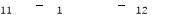xË†(y) =(10)eâˆ’y/Î²[f21(y) + Î¶2] + ey/Î²[âˆ’f22(y) + Î¶2]

where

f11(y) = f12

(âˆ’y) = sin(Î³/Î²) Im E( âˆ’y+ jÎ³)âˆ’Si(Î³/Î²) + Ï€1Î²2

âˆ’y+jÎ³

âˆ’cos(Î³/Î²) Â ReÂ  E1(Î²

+ Ci(Î³/Î²) ,(11)

f(y) = âˆ’f

1âˆ’y+ jÎ³(âˆ’y) = âˆ’ sin(Î³/Î²) Re E()+ Ci(Î³/Î²)

2122Î³1Î²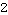1âˆ’y+jÎ³Ï€

âˆ’Î³cos(Î³/Î²) Â ImÂ  E1(Î²

âˆ’Si(Î³/Î²) + 2

,(12)

Î¶1 = lim f12

yâ†’âˆž

(y) = sin(Î³/Î²) âˆ’Si(Î³/Î²) + Ï€ âˆ’cos(Î³/Î²)Ci(Î³/Î²), and(13)Î¶= lim f

11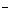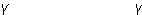(y) =sin(Î³/Î²)Ci(Î³/Î²) +cos(Î³/Î²)

Ï€Si(Î³/Î²) +

(14)

222

yâ†’âˆž

In the equations above, j= âˆšâˆ’1, Im{Â·}and Re{Â·}are the imaginary and real parts, respectively, of a complex argument, and E1(Â·), Si(Â·) and Ci(Â·) are, respectively, the exponential, sine and cosine integral functions obtained as in .

1. Experimental Results

Extensive experimentations are carried out in order to study the performance of the proposed despeckling scheme. The results are compared with those of other existing despeckling schemes that use improved-Lee filtering , adaptive-wavelet shrinkage , and contourlet thresholding . Performance evaluation of the various despeckling schemes is conducted on synthetically-speckled and real ultrasound images. In the implementation of the proposed speckling scheme, the 5-level decomposition of the curvelet transform is applied. From the experimental observation, applying a higher level of decomposition of the curvelet transform does not lead to any improvement in the despeckling performance. Since the curvelet transform is a shift-variant transform, the cycle spinning  is performed on the observed noisy image to avoid any possible pseudo-Gibbs artifacts in the neighborhood of discontinuities. In the proposed despeckling scheme, only the detail curvelet coefficients are despeckled using the Bayesian shrinkage function in (10).

The peak signal-to-noise ratio (PSNR) is used as a quantitative measure to assess the despeckling performance of the various schemes when applied on synthetically-speckled images. Table I gives the PSNR values obtained when applying the various schemes on two synthetically-speckled images of size 512Ã-512, namely, Lenaand Boat. It is obviously seen from this table that, in all cases, the proposed despeckling scheme provides higher values of PSNR compared to that provided by the other schemes. To have a better insight on the despeckling performance of the various schemes, the results in Table 1 are visualized in Figure 1. It is obvious from this figure that the superiority of the proposed scheme over the other schemes is more evident when a higher level of speckle noise is introduced to the test images. In order to study the performances of the various despeckling schemes on real ultrasound images, two images obtained from  and shown in Figure 2 are used. Since the noise-free images cannot be made available, one can only give a subjective evaluation of the performance of the various despeckling schemes. From Figure 2, it is clearly seen that the schemes in  and  provide despeckled images that suffer from the presence of visually noticeable speckle noise. On the other hand, the scheme in  severely over-smooth the noisy images thus providing despeckled images in which some of the texture details are lost. However, the proposed despeckling scheme results in images with not only a significant reduction in the speckle noise but also a good preservation of the textures of the original images.

Table 1: The PSNR values obtained when applying the various despeckling schemes on Lenaand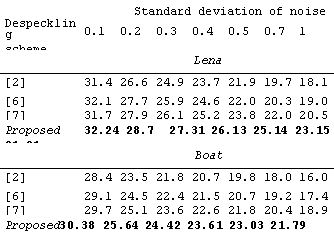Boatimages contaminated by speckle noise at different levels.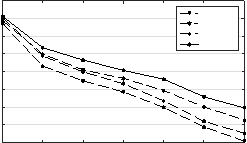34



32

30

Proposed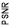28

26

24

22

20

18

0.10.20.30.40.50.71

Standard deviation of noise

(a)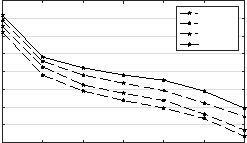32



30

28

Proposed26

24

22

20

18

16

0.10.20.30.40.50.71

Standard deviation of noise

(b)

Fig. 1: Quantitative comparison between the various despeckling schemes in terms of PSNR values:

(a) Lenaimage; (b) Boatimage.

1. Conclusion

In this paper, a new curvelet-based scheme for suppressing the speckle noise in ultrasound images has been developed in the framework of Bayesian estimation. The observed ultrasound image is first additively decomposed into noise-free and signal-dependant noise components. The Cauchy and twosided exponential distributions have been used as probabilistic models for the curvelet coefficients of the noise-free and signal-dependant noise components, respectively, of the ultrasound image. The proposed probabilistic models of the curvelet coefficients of an observed ultrasound image has been employed to formulate a Bayesian shrinkage function in order to obtain the estimates of the noise-free curvelet coefficients. A low-complexity realization of this shrinkage function has been employed. Experiments have been carried out on both synthetically-speckled and real ultrasound images in order to demonstrate the performance of the proposed despeckling scheme. In comparison with some other existing despeckling schemes, the results have shown that the proposed scheme provides higher PSNR values and gives well-despeckled images with better diagnostic details.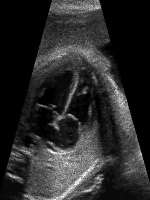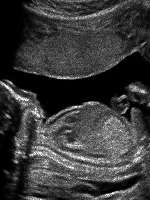1. (b)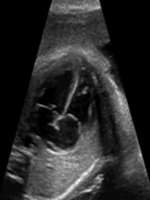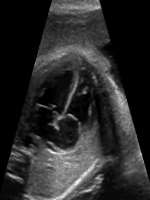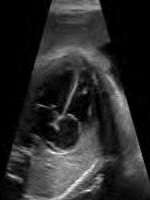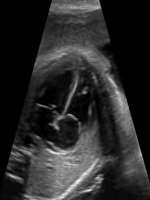(c)(d)(e)(f)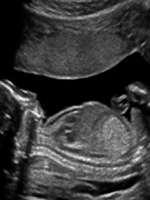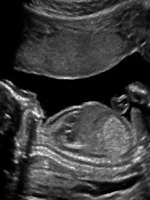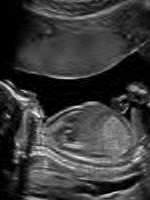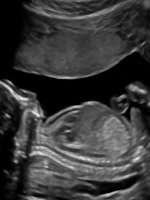(g)(h)(i)(j)

Fig. 2: Qualitative comparison between the various despeckling schemes. (a)(b) Noisy ultrasound images. Despeckled images obtained by applying the schemes in (c)(g)  ,(d)(h)  ,(e)(i)  and (f)(j) the proposed scheme.

References

1. Dhawan, A.P.: Medical image analysis. Volume 31. John Wiley & Sons (2011)
2. Loupas, T., McDicken, W., Allan, P.: Â An adaptive weighted median filter for speckle suppression in medical ultrasonic images. IEEE transactions on Circuits and Systems 36(1) (1989) 129-135
3. CoupÂ´e, P., Hellier, P., Kervrann, C., Barillot, C.: Nonlocal means-based speckle filtering for ultrasound images. IEEE transactions on image processing 18(10) (2009) 2221-2229
4. Sridhar, B., Reddy, K., Prasad, A.: An unsupervisory qualitative image enhancement using adaptive morphological bilateral filter for medical images. International Journal of Computer Applications 10(2i) (2014) 1
5. Abd-Elmoniem, K.Z., Youssef, A.B., Kadah, Y.M.: Real-time speckle reduction and coherence enhancement in ultrasound imaging via nonlinear anisotropic diffusion. IEEE Transactions on Biomedical Engineering 49(9) (2002) 997-1014
6. Swamy, M., Bhuiyan, M., Ahmad, M.: Spatially adaptive thresholding in wavelet domain for despeckling of ultrasound images. IET Image Process 3(3) (2009) 147-162
7. Hiremath, P., Akkasaligar, P.T., Badiger, S.: Speckle reducing contourlet transform for medical ultrasound images. Int J Compt Inf Engg 4(4) (2010) 284-291
8. Jian, Z., Yu, Z., Yu, L., Rao, B., Chen, Z., Tromberg, B.J.: Speckle attenuation in optical coherence tomography by curvelet shrinkage. Optics letters 34(10) (2009) 1516-1518
9. Deng, C., Wang, S., Sun, H., Cao, H.: Multiplicative spread spectrum watermarks detection performance analysis in curvelet domain. In: 2009 International Conference on E-Business and Information System Security. (2009)
10. Damseh, R.R., Ahmad, M.O.: A low-complexity mmse bayesian estimator for suppression of speckle in sar images. In: Circuits and Systems (ISCAS), 2016 IEEE International Symposium on, IEEE (2016) 1002-1005
11. Temizel, A., Vlachos, T., Visioprime, W.: Wavelet domain image resolution enhancement using cycle-spinning. Electronics Letters 41(3) (2005) 119-121
12. Siemens Â Healthineers:Â  https://www.healthcare.siemens.com/ultrasound. Accessed: Â 2017-01-06.

View all

## DMCA / Removal Request

If you are the original writer of this essay and no longer wish to have your work published on UKEssays.com then please:

Related Services

Related Lectures